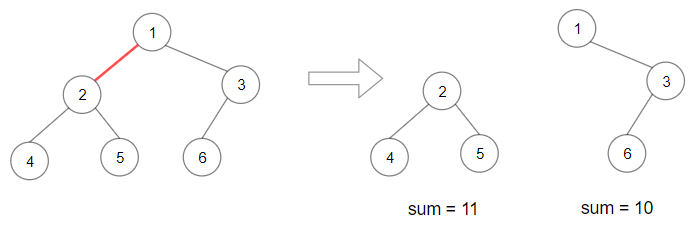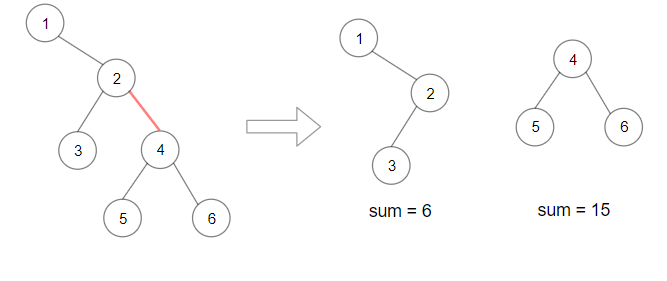# LeetCode Weekly Contest 174

https://leetcode.com/contest/weekly-contest-174/

weekly contest在每周周六9.30 p.m. biweekly contest在每两周周六9.30 a.m.，我真没那么多时间再来刷这些没什么意思的题了，反正也找不到工作（当然有任何intern机会还请联系我……我真的现在看现在的就业情况好焦虑啊）

## The K Weakest Rows in a Matrix

https://leetcode.com/contest/weekly-contest-174/problems/the-k-weakest-rows-in-a-matrix/

Given a m * n matrix mat of ones (representing soldiers) and zeros (representing civilians), return the indexes of the k weakest rows in the matrix ordered from the weakest to the strongest.

A row i is weaker than row j, if the number of soldiers in row i is less than the number of soldiers in row j, or they have the same number of soldiers but i is less than j. Soldiers are always stand in the frontier of a row, that is, always ones may appear first and then zeros.

Example 1:

Example 2:

Constraints:

1. m == mat.length
2. n == mat[i].length
3. 2 <= n, m <= 100
4. 1 <= k <= m
5. matrix[i][j] is either 0 or 1.

## Reduce Array Size to The Half

https://leetcode.com/contest/weekly-contest-174/problems/reduce-array-size-to-the-half/

Given an array arr. You can choose a set of integers and remove all the occurrences of these integers in the array.

Return the minimum size of the set so that at least half of the integers of the array are removed.

Example 1:

Example 2:

Example 3:

Example 4:

Example 5:

Constraints:

1. 1 <= arr.length <= 10^5
2. arr.length is even.
3. 1 <= arr[i] <= 10^5

## Maximum Product of Splitted Binary Tree

Given a binary tree root. Split the binary tree into two subtrees by removing 1 edge such that the product of the sums of the subtrees are maximized.

Since the answer may be too large, return it modulo 10^9 + 7.

Example 1:Example 2:Example 3:

Example 4:

Constraints:

1. Each tree has at most 50000 nodes and at least 2 nodes.
2. Each node’s value is between [1, 10000].

## Jump Game V

https://leetcode.com/contest/weekly-contest-174/problems/jump-game-v/

Given an array of integers arr and an integer d. In one step you can jump from index i to index:

• i + x where: i + x < arr.length and 0 < x <= d.
• i - x where: i - x >= 0 and 0 < x <= d.
In addition, you can only jump from index i to index j if arr[i] > arr[j] and arr[i] > arr[k] for all indices k between i and j (More formally min(i, j) < k < max(i, j)).

You can choose any index of the array and start jumping. Return the maximum number of indices you can visit.

Notice that you can not jump outside of the array at any time.

Example 1:Example 2:

Example 3:

Example 4:

Example 5:

Constraints:

1. 1 <= arr.length <= 1000
2. 1 <= arr[i] <= 10^5
3. 1 <= d <= arr.length